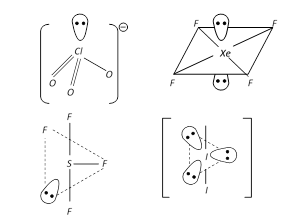Courses

# Test: Bond Parameters

## 20 Questions MCQ Test Chemistry Class 11 | Test: Bond Parameters

Description
This mock test of Test: Bond Parameters for Class 11 helps you for every Class 11 entrance exam. This contains 20 Multiple Choice Questions for Class 11 Test: Bond Parameters (mcq) to study with solutions a complete question bank. The solved questions answers in this Test: Bond Parameters quiz give you a good mix of easy questions and tough questions. Class 11 students definitely take this Test: Bond Parameters exercise for a better result in the exam. You can find other Test: Bond Parameters extra questions, long questions & short questions for Class 11 on EduRev as well by searching above.
QUESTION: 1

### Two atoms are said to be bonded when:

Solution:

Attractive forces tend to bring two atoms close to each other whereas repulsive forces tend to move them away. In hydrogen, the magnitude of the new attractive forces is greater than that of new repulsive forces. As a result, two atoms come close to each other and potential energy decreases. The atoms approach each other until the equilibrium stage is reached where the net force of attraction balances the force of repulsion and system acquires minimum energy.

QUESTION: 2

### The decreasing order of the repulsive interactions between various electron pairs is:

Solution:

The bond pair of electrons is shared by two atoms whereas the lone pair of electrons is only under the influence of central atom.

So the electron cloud of lone pair occupies more space as compared to the bond pair.

This causes greater repulsion between the lone pairs as compared to lone pair-bond pair and bond pair-bond pair interactions.

QUESTION: 3

### Valence bond theory was introduced by:

Solution:

Valence bond theory was introduced by Heitler and London (1927) and further work was done by Pauling and others.

In the valence bond (VB) theory, proposed in large part by the American scientists Linus Pauling and John C. Slater, bonding is accounted for in terms of hybridized orbitals of the metal ion, which is assumed to possess a particular number of vacant orbitals available for coordinate bonding that equals its coordination number.

QUESTION: 4

Which molecule has a bent shape?

Solution:

CO2 and BeH2 possess linear geometry as well as linear shape , while H2O possess traversal geometry and bent shape.NF3 possess traversal geometry and trigonal pyramidal shape . Thus correct option is (c)

QUESTION: 5

The s-orbital does not show preference to any direction because

Solution:

The s-orbital is spherically symmetric in shape so it does not show preference to any direction. It is the same from all the directions.

QUESTION: 6

The number of lone pairs of electrons on SF4, CF4 and XeF4 are:

Solution:

SF4 molecule has 1 lone pair of electrons, CF4 has no lone pair of electrons and XeF4 has 2 lone pair of electrons respectively.

QUESTION: 7

Pick out the pair of species having identical shapes for both the molecules.

Solution:

Both XeF& CO2 have linear structures. Both are actually linear Tri-atomic molecules.

QUESTION: 8

Among the following species octahedral shape is found in:

Solution:

SF6 has an octahedral geometry.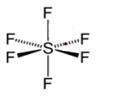QUESTION: 9

Which molecule has a trigonal pyramidal shape?

Solution:

AB3E: trigonal pyramidal (central atom + 3 outer atoms make a pyramid)
→ start with AB4 molecule (tetrahedral) and replace a B atom w/ lone pair
→ lone pair electrons push bonding electrons away
→ bond angles are now less than 109.5°

QUESTION: 10

Which of the following orbitals give a zero overlap?

Solution: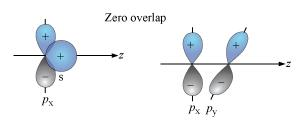QUESTION: 11

Among the following molecules, the molecule with trigonal planar geometry is:

Solution:

(a) BF₃ ⇒ 3bp + 0/p ⇒ sp³ -hybridisation and triagonal planar geometry.
(b) IF₃ ⇒ 3bp + 2/p ⇒ sp³d -hybridisation and T shape.
(c) NH₃ ⇒ 3bp + 1/p ⇒ sp³ -hybridisation and pyraminal geometry.
(d) PH₃ ⇒ 3bp + 1/p ⇒ sp³ -hybridisation and pyraminal geometry.

QUESTION: 12

A pi-bond is formed by the overlap of:

Solution:

pi-bond is always formed by sidewise overlapping of p – p orbitals in sidewise manner.

QUESTION: 13

Which of the following has a square pyramidal shape?

Solution:

Br-atom has configuration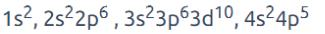To get pentavalency, two of the p-orbitals are unpaired and electrons are shifted to 4d-orbitals.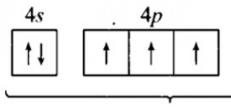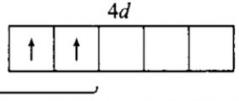In this excited state, sp3d2-hybridisation occurs giving octahedral structure. Five positions are occupied by F atoms forming sigma bonds with hybrid bonds and one position occupied by lone pair, i.e., the molecule as a square pyramidal shape.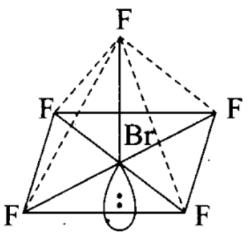QUESTION: 14

According to the VSEPR theory, the geometry and shape of the molecule depends upon:

Solution:

For geometry and shape of a molecule, both bond pair and lone pair of electron in valence shell of central atom.

QUESTION: 15

The statement that is true about H2O molecule is:

Solution:

Central atom: O

At. no. = 8,

No. of valence electrons in oxygen is 6

Lewis dot structure of O atom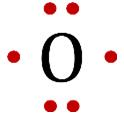No. of electrons required for completing the octet = 8 - 6 =2

Atoms attached to the central atom: two H atoms

At. no. of H = 1

H atoms need one electron each for getting fully-filled K shells.

Representation of Lewis dot structures of H2O, which satisfies these conditions: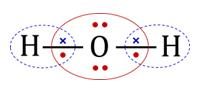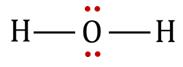No. of valence shell electron pairs on the central atom O, after forming two covalent bonds with two H atoms = 4.

Geometry of 4 pairs, as predicted by VSEPR theory = Tetrahedral.

No. of lone pairs = Two.

Type, according to VSEPR theory:

So, geometry of pairs and geometry of atoms are different.

The geometry of atoms is derived from the geometry of pairs. At two of the four corners of the tetrahedron, there are no atoms.

Diagrams showing the geometry of 2 H atoms and two lone pairs: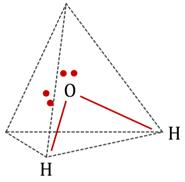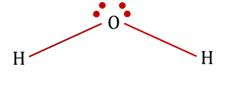Geometry of atoms in H2O is V-shaped. i.e. H2O molecule has a bent geometry

QUESTION: 16

Valence Bond Theory was developed in the year

Solution:

The valence bond (VB) theory of bonding was mainly developed by Walter Heitler and Fritz London in 1927, and later modified by Linus Pauling to take bond direction into account. The VB approach concentrates on forming bonds in localized orbitals between pairs of atoms, and hence retains the simple idea of Lewis structures and electron pairs.

QUESTION: 17

Among the following species linear shape is found in:

Solution: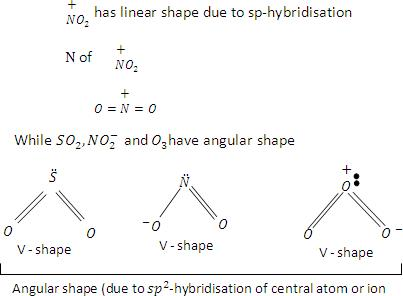QUESTION: 18

Which of the following molecule doesn’t have a lone pair?

Solution:

BeCl2 has no lone pairs on the beryllium. Thus, the electrons on the chlorides willtry to stay far apart from each other, since their corresponding electrons repel each other (while experiencing no deflection from electrons on a central atom). Thus themolecule is linear in shape.

QUESTION: 19

Among the following species the pair that have V-shaped geometry is:

Solution:

Both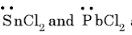are bent shape molecule.

QUESTION: 20

Among the following compounds the maximum number of lone pair is present on the central atom of:

Solution: